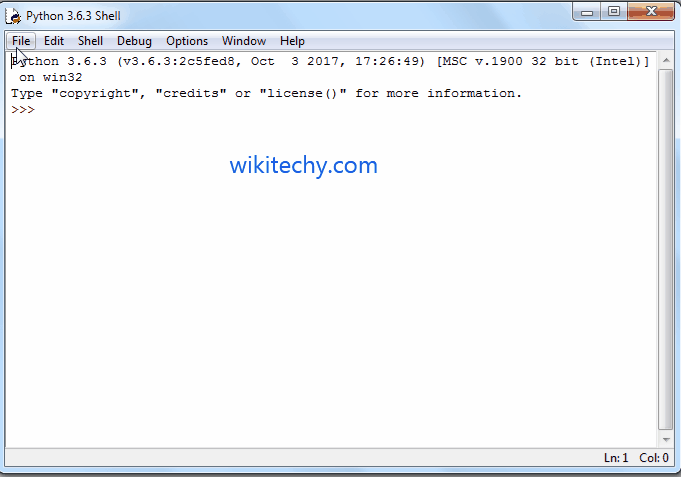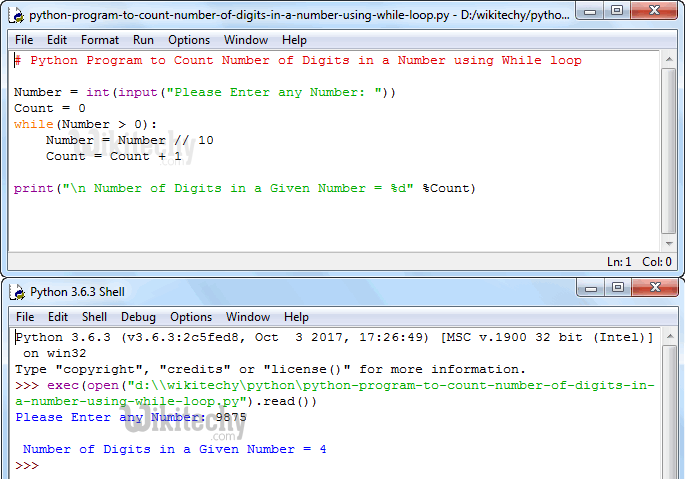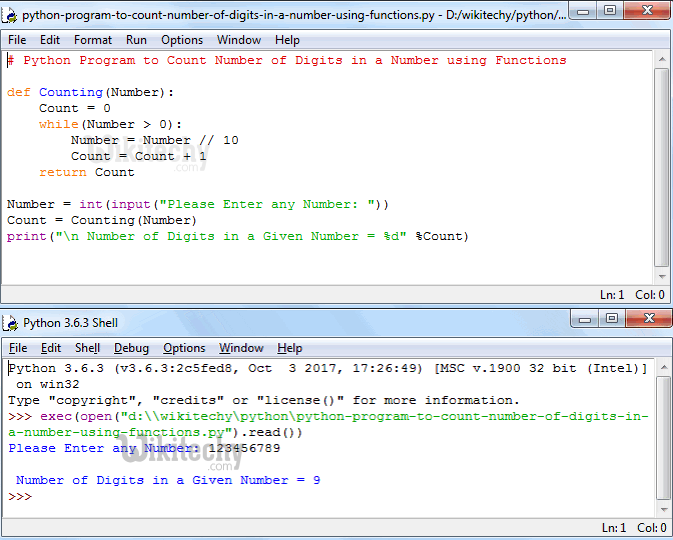# python tutorial - Python Program to Count Number of Digits in a Number - learn python - python programming

• To write a Python Program to Count Number of Digits in a Number using While Loop, Functions and RecursionLearn Python - Python tutorial - Python Program to Count Number of Digits in a Number - Python examples - Python programs

## Python Program to Count Number of Digits in a Number using While Loop

• This program allows the user to enter any positive integer and then it will divide the given number into individual digits and count those individual digits using Python While Loop.

## OutputLearn Python - Python tutorial - Python Program to Count Number of Digits in a Number using While Loop - Python examples - Python programs

## Analysis

• This program allows the user to enter any positive integer and then, that number is assigned to the Number variable.
• Next, Condition in the While loop will make sure that, the given number is greater than 0 (Means Positive integer and greater than 0)
• User Entered value: Number = 9875 and Count = 0

## First Iteration

Number = Number // 10
Number = 9875 //10
Number = 987
Count = Count + 1
Count = 0 + 1
Count = 1

## Second Iteration

From the first Iteration the values of both Number and Count has been changed as: Number = 987 and Count = 1
Number = Number // 10
Number = 987 // 10
Number = 98
Count = Count + 1
Count = 1 + 1
Count = 2

## Third Iteration

From the Third Iteration the values of both Number and Count has been changed as: Number = 98 and Count = 2
Number = Number // 10
Number = 98 // 10
Number = 9
Count = Count + 1
Count = 2 + 1
Count = 3

## Fourth Iteration

From the Fourth Iteration the values of both Number and Count has been changed as: Number = 9 and Count = 3
Number = Number // 10
Number = 9 // 10
Number = 0
Count = Count + 1
Count = 3 + 1
Count = 4

## Python Program to Count Number of Digits in a Number Using Functions

• This program allows the user to enter any positive integer and then it will divide the given number into individual digits and counting those individual digits using Functions.

• OR

## OutputLearn Python - Python tutorial - Python Program to Count Number of Digits in a Number Using Functions - Python examples - Python programs

## Analysis

• When the compiler reaches to Counting (Number) line python program then the compiler will immediately jump to below function:
• We already explained LOGIC in above example. Please refer Python Program to Find Count of the Digits of a Given Number using While Loop Analysis section.
• Last line ends with return Count Statement.

## Python Program to Count Number of Digits in a Number Using Recursion

• This program allows the user to enter any positive integer and then it will divide the given number into individual digits and count those individual digits using Recursion.

## OutputLearn Python - Python tutorial - Python Program to Count Number of Digits in a Number Using Recursion - Python examples - Python programs

## Analysis

• In the Counting (Number) function definition,
• Below statement will help to call the function Recursively with updated value.
• If you miss this statement then, after completing the first line it will terminate. For example,
• Number = 1234
• Then the Output will be 1